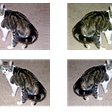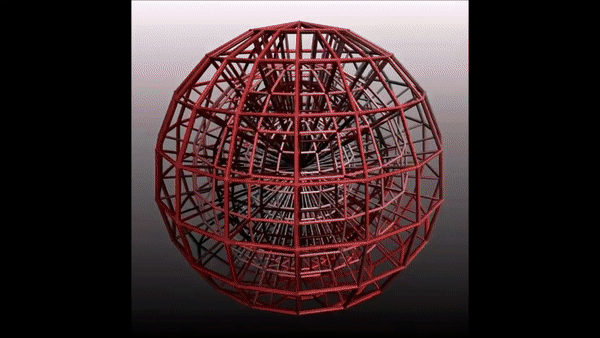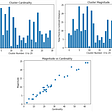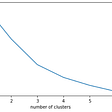# What is K-means Clustering?

K-means clustering is a type of unsupervised learning, which is used when you have unlabeled data (data without defined categories or groups). The goal of this algorithm is to find groups in the data, with the number of groups represented by the variable K

The results of the K-means clustering algorithm are:

The centroids of the K clusters, which can be used to label new data

Labels for the training data (each data point is assigned to a single cluster)

# Where k-means clustering algorithm is used?

k-mean clustering algorithm is used in Machine Learning models where we have to do unsupervised learning with improper historical data , so for that case we use k-means clustering algorithm.

# What are the basic steps for K-means clustering?

• Step 1: Choose the number of clusters k.
• Step 2: Select k random points from the data as centroids.
• Step 3: Assign all the points to the closest cluster centroid.
• Step 4: Re-compute the centroids of newly formed clusters.
• Step 5: Repeat steps 3 and 4.

How does k-means clustering work?

The k-means clustering algorithm attempts to split a given anonymous data set (a set containing no information as to class identity) into a fixed number (k) of clusters.

Initially k number of so called centroids are chosen. A centroid is a data point (imaginary or real) at the center of a cluster. In Praat each centroid is an existing data point in the given input data set, picked at random, such that all centroids are unique (that is, for all centroids ci and cj, cicj). These centroids are used to train a KNN Classifier. The resulting classifier is used to classify (using k = 1) the data and thereby produce an initial randomized set of clusters. Each centroid is thereafter set to the arithmetic mean of the cluster it defines. The process of classification and centroid adjustment is repeated until the values of the centroids stabilize. The final centroids will be used to produce the final classification/clustering of the input data, effectively turning the set of initially anonymous data points into a set of data points, each with a class identity.

# Algorithm :

Κ-means clustering algorithm inputs are the number of clusters Κ and the data set. The algorithm starts with initial estimates for the Κ centroids, which can either be randomly generated or randomly selected from the data set. The algorithm then iterates between two steps:

1. Data assignment step:

Each centroid defines one of the clusters. In this step, each data point based on the squared Euclidean distance is assigned to its nearest centroid. If 𝑐𝑖ci is the collection of centroids in set C, then each data point x is assigned to a cluster based on

min𝑐𝑖∈𝐶𝑑𝑖𝑠𝑡(𝑐𝑖,𝑥)2minci∈Cdist(ci,x)2

where dist( · ) is the standard (L2) Euclidean distance.

2. Centroid update step:

Centroids are recomputed by taking the mean of all data points assigned to that centroid’s cluster.

The algorithm iterates between step one and two until a stopping criteria is met (no data points change clusters, the sum of the distances is minimized, or some maximum number of iterations is reached).

This algorithm may converge on a local optimum. Assessing more than one run of the algorithm with randomized starting centroids may give a better outcome.

Choosing K

If the true label is not known in advance, then K-Means clustering can be evaluated using Elbow Criterion , Silhouette Coefficient , cross-validation, information criteria, the information theoretic jump method, and the G-means algorithm. .

Elbow Criterion Method:

The idea behind elbow method is to run k-means clustering on a given dataset for a range of values of k (e.g k=1 to 10), for each value of k, calculate sum of squared errors (SSE).

Calculate the mean distance between data points and their cluster centroid. Increasing the number of clusters(K) will always reduce the distance to data points, thus decrease this metric, to the extreme of reaching zero when K is as same as the number of data points. So the goal is to choose a small value of k that still has a low SSE.

We run the algorithm for different values of K(say K = 10 to 1) and plot the K values against SSE(Sum of Squared Errors). And select the value of K for the elbow point.

Silhouette Coefficient Method:

A higher Silhouette Coefficient score relates to a model with better-defined clusters. The Silhouette Coefficient is defined for each sample and is composed of two scores:

• The mean distance between a sample and all other points in the same class.
• The mean distance between a sample and all other points in the next nearest cluster.

The Silhouette Coefficient is for a single sample is then given as:

𝑠=𝑏−𝑎𝑚𝑎𝑥(𝑎,𝑏)s=b−amax(a,b)

To find the optimal value of k for KMeans, loop through 1..n for n_clusters in KMeans and calculate Silhouette Coefficient for each sample.

A higher Silhouette Coefficient indicates that the object is well matched to its own cluster and poorly matched to neighboring clusters.

K-Means algorithm uses Eucledean Distance, other popular distance metrics in Machine Learning are:

1. Cosine distance : It determines the cosine of the angle between the point vectors of the two points in the n dimensional space. Closer the point vectors are by angle, the higher is the Cosine Similarity

cos𝜃=𝑎→.𝑏→∥𝑎→∥∥𝑏→∥=∑𝑛𝑖=1𝑎𝑖𝑏𝑖∑𝑛𝑖=1𝑎2𝑖∑𝑛𝑖=1𝑏2𝑖⎯⎯⎯⎯⎯⎯⎯⎯⎯⎯⎯⎯√⎯⎯⎯⎯⎯⎯⎯⎯⎯⎯⎯⎯⎯⎯⎯⎯⎯⎯⎯⎯⎯⎯⎯⎯⎯⎯⎯⎯√cos⁡θ=a→.b→∥a→∥∥b→∥=∑i=1naibi∑i=1nai2∑i=1nbi2

where 𝑎→.𝑏→=∑𝑛𝑖=1𝑎𝑖𝑏𝑖=𝑎1𝑏1+𝑎2𝑏2+…+𝑎𝑛𝑏𝑛a→.b→=∑i=1naibi=a1b1+a2b2+…+anbn

1. Manhattan distance : is the total sum of the difference between the x-coordinates and y-coordinates.

𝑀𝑎𝑛ℎ𝑎𝑡𝑡𝑎𝑛𝐷𝑖𝑠𝑡𝑎𝑛𝑐𝑒=|𝑥1–𝑥2|+|𝑦1–𝑦2|ManhattanDistance=|x1–x2|+|y1–y2|

Both the RMSE and the MAE are ways to measure the distance between two vectors: the vector of predictions and the vector of target values. Various distance measures, or norms, are possible:

• Computing the root of a sum of squares (RMSE) corresponds to the Euclidian norm: it is the notion of distance you are familiar with. It is also called the ℓ2 norm(…)
• Computing the sum of absolutes (MAE) corresponds to the ℓ1 norm,(…). It is sometimes called the Manhattan norm because it measures the distance between two points in a city if you can only travel along orthogonal city blocks.

# 1. Spam filter

What the problem is: Spam emails are at best an annoying part of modern-day marketing techniques, and at worst, an example of people phishing for your personal data. To avoid getting these emails in your main inbox, email companies use algorithms. The purpose of these algorithms is to flag an email as spam correctly or not.

How clustering works: K-Means clustering techniques have proven to be an effective way of identifying spam. The way that it works is by looking at the different sections of the email (header, sender, and content). The data is then grouped.

These groups can then be classified to identify which are spam. Including clustering in the classification process improves the accuracy of the filter to 97%. This is excellent news for people who want to be sure they’re not missing out on your favorite newsletters and offers.

# 2. Classifying network traffic

Imagine you want to understand the different types of traffic coming to your website. You are particularly interested in understanding which traffic is spam or coming from bots.

What the problem is: As more and more services begin to use APIs on your application, or as your website grows, it is important you know where the traffic is coming from. For example, you want to be able to block harmful traffic and double down on areas driving growth. However, it is hard to know which is which when it comes to classifying the traffic.

How clustering works: K-means clustering is used to group together characteristics of the traffic sources. When the clusters are created, you can then classify the traffic types. The process is faster and more accurate than the previous Autoclass method. By having precise information on traffic sources, you are able to grow your site and plan capacity effectively.

# 3. Fantasy Football and Sports

Ok so up until this point we have looked into different business problems and how clustering algorithms have been applied to solve them. But now for the critical issues — fantasy football!

What is the problem: Whom should you have in your team? Which players are going to perform best for your team and allow you to beat the competition? The challenge at the start of the season is that there is very little if any data available to help you identify the winning players.

How clustering works: When there is little performance data available to train your model on, you have an advantage for unsupervised learning. In this type of machine learning problem, you can find similar players using some of their characteristics. This has been done using K-Means clustering. Ultimately this means you can get a better team more quickly at the start of the year, giving you an advantage.

# Drawbacks

Kmeans algorithm is good in capturing structure of the data if clusters have a spherical-like shape. It always try to construct a nice spherical shape around the centroid. That means, the minute the clusters have a complicated geometric shapes, kmeans does a poor job in clustering the data. We’ll illustrate three cases where kmeans will not perform well.

First, kmeans algorithm doesn’t let data points that are far-away from each other share the same cluster even though they obviously belong to the same cluster. Below is an example of data points on two different horizontal lines that illustrates how kmeans tries to group half of the data points of each horizontal lines together.

--

--

--

## More from Aakash Bhardwaj

Love podcasts or audiobooks? Learn on the go with our new app.

## What’s next to improve the model accuracy of Convolutional Neural Networks (CNNs)## Deep Learning #3: More on CNNs & Handling Overfitting## Zonked on Vicodin in the Presidential Race: Generating Fake Headlines With Markov Chains## SphereFace Loss-Deep Face Recognition## Creating Mo-Cap Facial Animations of Virtual Characters with Deep Learning## LawBERT: a Legal Domain-Specific BERT?## Remove objects from photos — — Easy to use Image Inpainting## K-Means Clustering on Country Dataset## K-Means Clustering From Scratch-with Manual Similarity Measure## Unleashing Unsupervised Learning | Dataloop Blog## The intuition behind K-means clustering technique